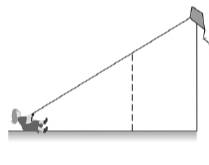Chapter 5.2, Problem 30EElementary Geometry For College St...

7th Edition
Alexander + 2 others
ISBN: 9781337614085

Solutions

Chapter
SectionElementary Geometry For College St...

7th Edition
Alexander + 2 others
ISBN: 9781337614085
Textbook Problem

In Exercise 29 to 32, use the fact that triangles are similar.With 100 ft of string out, a kite is 64 ft above ground level. When the girl flying the kite pulls in 40 ft of string, the angle formed by the string and the ground does not change. What is the height of the kite above the ground after the 40 ft of string have been taken in?To determine

To calculate:

The height of the kite above the ground after the 40ft of string.

Explanation

Given:

With 100ft of string out, a kite is 64ft above ground level. When the girl flying the kite pulls in 40ft of string, the angle formed by the string and the ground does not change.

Definition Used:

Similarity between Two polygons:

Two polygons are similar if and only if when all pairs of corresponding angles are congruent and all pairs of corresponding sides are proportional.

Calculation:

Here, AE¯ represents the original string, and CE¯ represents the string after 40ft.

According to the definition of segment-addition postulate, AE=AC+CE.

It is given that the length of the original string is AE=100ft.

The girl flying the kitepulls the string at AC=40ft.

So,

Still sussing out bartleby?

Check out a sample textbook solution.

See a sample solution

The Solution to Your Study Problems

Bartleby provides explanations to thousands of textbook problems written by our experts, many with advanced degrees!

Get Started

In Problems 7-20, find each limit, if it exists.

Mathematical Applications for the Management, Life, and Social Sciences

Fill in each blank: 168ft=in.

Elementary Technical Mathematics

Express the function in the form f g. 45. F(x)=x31+x3

Single Variable Calculus: Early Transcendentals, Volume I

Proof Prove theorem 11.6.

Calculus (MindTap Course List)